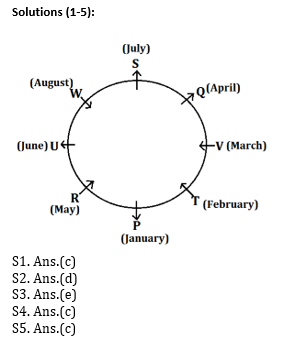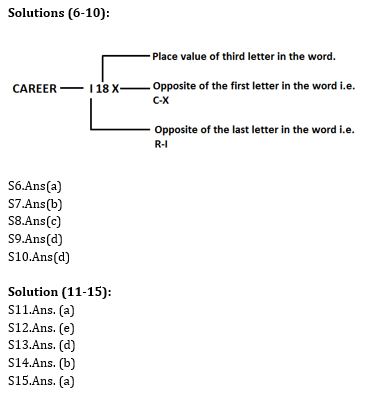# Reasoning Ability Quiz For Bank Mains Exams 2021- 11th January

Directions (1-5): Study the following information carefully and answer the question given below-
Eight persons P, Q, R, S, T, U, V and W are sitting around a circular table. Four of them faces toward center while the rest of them faces opposite to center. Each of them was born in a different month of the same year starting from January to August but not necessarily in the same order.
T was born in February. S who is the second youngest sits opposite to P. The persons who were born in June and March are sitting opposite to each other. R who was born in the month of May is an immediate neighbour of P. The person who was born in April sits third to the right of U. W is the youngest and sits opposite to T. V faces opposite direction of U. Both the immediate neighbours of P are facing towards the center. Q sits second to the right of T. T sits third to the right of S who faces outside. S is an immediate neighbour of Q. W faces towards the center. V is older than U. Only one person sits between S and U who is not immediate neighbor of T.

Q1. Who among the following was born in the month of March?
(a) Q
(b) R
(c) V
(d) P
(e) W

Q2. Who among the following sits third to the left of the person who was born in May?
(a) P
(b) R
(c) T
(d) S
(e) W

Q3. Who among the following is the third youngest person?
(a) Q
(b) R
(c) T
(d) P
(e) U

Q4. How many persons facing towards the centre are older than Q?
(a) Three
(b) One
(c) Two
(d) Four
(e) None of these

Q5. The person sitting third to the right of P was born in which of the following months?
(a) January
(b) February
(c) August
(d) April
(e) None of these

Directions (6-10): Study the following information carefully to answer the given questions. In a certain code language:-
“Ordinary Through Political Career” is written as ‘B4L S18G O12K I18X’.
“Leader Earlier Allegiance” is written as ‘I1O I18V V12Z’.
“Advantage Introduced Variables” is written as ‘V22Z W20R H18E’.

Q6. “Chemistry” will be coded as?
(a) B5X
(b) B4X
(c) X5B
(d) X6B
(e) None of these

Q7. “Random” will be coded as?
(a) W1X
(b) N14I
(c) X18W
(d) I15N
(e) None of these

Q8. What is the code for “Political”?
(a) I18X
(b) W20R
(c) O12K
(d) I1O
(e) None of these

Q9. Which will be the code for ‘Evening’?
(a) R5C
(b) T8U
(c) S9K
(d) T5V
(e) None of these

Q10. What is the code for “Developer”?
(a) W22I
(b) I20R
(c) U22L
(d) I22W
(e) None of these

Directions (11-15): In the following questions, the symbols @, #, %, \$ and * are used with the following meaning as illustrated below-
P@Q – P is neither greater than nor equal to Q
P%Q – P is neither smaller than nor equal to Q
P#Q – P is not greater than Q
P\$Q – P is not smaller than Q
P*Q- P is neither smaller than nor greater than Q
Now in each of the following questions assuming the given statement to be true, find which of the two conclusions I and II given below them is/are definitely true and give your answer accordingly.

Q11. Statements: – Z@Y, Y%X, X*W, W\$V
Conclusions: – (I) Y%V (II) Z%V
(a) Only conclusion I follows
(b) Only conclusion II follows
(c) Either conclusion I or II follows
(d) Neither conclusion I nor II follow
(e) Both conclusion I and II follows

Q12. Statements: – Z%Y, Y*X, X#W, W*V
Conclusions: – (I) Z%X (II) V\$Y
(a) Only conclusion I follows
(b) Only conclusion II follows
(c) Either conclusion I or II follows
(d) Neither conclusion I nor II follow
(e) Both conclusion I and II follows

Q13. Statements: – Z*Y, Y\$X, X#W, W@V
Conclusions: – (I) V*Z (II) X%Z
(a) Only conclusion I follows
(b) Only conclusion II follows
(c) Either conclusion I or II follows
(d) Neither conclusion I nor II follow
(e) Both conclusion I and II follows

Q14. Statements: – Z\$Y, Y\$X, X*W, W@V
Conclusions: – (I) Z\$V (II) V%X
(a) Only conclusion I follows
(b) Only conclusion II follows
(c) Either conclusion I or II follows
(d) Neither conclusion I nor II follow
(e) Both conclusion I and II follows

Q15. Statements: – Z%V, V@X, X%Y, Y*W
Conclusions: – (I) X%W (II) Y@Z
(a) Only conclusion I follows
(b) Only conclusion II follows
(c) Either conclusion I or II follows
(d) Neither conclusion I nor II follow
(e) Both conclusion I and II follows

SolutionsPractice with Online Test Series for Bank Mains 2021: